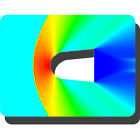Aerodynamics Tool Free

All Android applications categories

All Android games categories# Aerodynamics Tool Free

122 8

8 Users
rating

## Screenshots

Description

1. Basic Aerodynamic Calculator- Calculation of Dynamic Pressure, Reynolds Number, Force and Moment Coefficient for Incompressible and Compressible flow.

2. Isentropic Relations - If any one of the variables, M, Po/P, To/T, Rho/Rh or A/A* is known the remaining variable values can be computed.

3. Normal Shock- Select any known variable from list, M1, P2/P1, T2/T1, M2, rho2/rho1, Po2/Po1, and Po2/P1 and user can calculate remaining variable values. Indices 1 and 2 are for before shock and after shock respectively.

4. Oblique Shock - Theta-Beta-Mech number relation. If values of any two variable known then user can calculate value of remaining one.

5. Vortex Lattice Method - Vortex Lattice Method is a method used to calculate lift coefficient of Finite Wing at low speed. It gives fairly good CL estimation for Swept wing compared to Vortex Panel Method. This tool is helpful for primary aerodynamic design iterations. The method presented here uses 'n' spanwise divisions and one chordwise division of wing. The final output is slope of CL vs Alpha curve and it is CL per degree angle of attack.

6. Prandtl-Meyer expansion wave relations - Compute v1 from M1 (Mach Number before expansion wave). v is Prandtl-Meyer function. Add deflection angle theta to v1 to get v2. From v2 compute M2 (Mach Number after expansion wave).

7. Pitot Tube - Divided in two categories. One for Incompressible Flow and Other for Compressible Flow. In case of Incompressible flow, user can input height of Pitot Tube column in Meters of Water, Mercury or Any known fluid to calculate free stream velocity. The compressible flow case takes inputs for Total Pressure and Static Pressure measured by Pitot Tube and calculates Free Stream Mach Number. User need not to worry about Subsonic or Supersonic flows. These are identified and solved accordingly.

8. Y Plus or Y+ - It calculates first cell height of grid near wall to resolve boundary layer.

9. Check points for Inputs to avoid wrong inputs and flash suggestions if any.

If you like this, please consider buying ad-free version which can take any value of Specific Heat Ratio (Gamma) and not fixed to 1.4.

If found any Bug or wrong output, please mail me regarding issue

Before giving negative rating for pop-up ads please consider that I have tried to keep ads when user finishes using any one of the utility of this app and not at the launch of utility which is more objectionable.